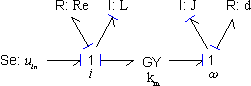﻿ 20-sim webhelp > Modeling Tutorial > Bond Graphs > causality

# causality

Causal analysis is the determination of the direction of the efforts and flows in a bond graph model. The result is a causal bond graph which can be considered as a compact block diagram. From causal bond graph we can directly derive an equivalent block diagram. In 20-sim causality is assigned automatically. To create a causal bond graph 20-sim will perform the following steps.

 1 Apply causality for all elements with fixed causality (Se,Sf,Mse,MSf,user defined models), i.e. assign a causal stroke to all bonds connected to elements with fixed causality.
 2 Apply,as much as possible, causality for elements that have a constraint causality (
 3 Apply causality for an arbitrary element with preferred causality (C,I,user defined models). If possible, let this be the preferred causality. Now iterate step 2 and 3 as far as possible.
 4 Apply causality for an arbitrary element with indifferent causality (R,user defined models). If this causality is not fixed, choose a causality arbitrarily. Now iterate step 2, 3 and 4 as far as possible.

In many cases, the bond graph model is causal after step 2. However, sometimes as causal conflict occurs. This means the model is not correct arithmetically. Often this points out an ill-defined model. Redefinition of the ideal physical model will solve the problem. Sometimes the model is only causal after step 4. This means the model contains an algebraic loop. Models with algebraic loops are generally hard to simulate.

## Example

Causal assignment will be illustrated by an example. We will manually create a causal bond graph out of the following model: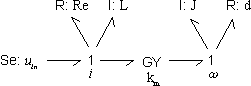1 Apply fixed causality: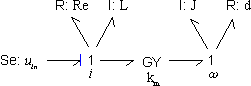2 Apply constraint causality. This step is not yet applicable.
 3 Apply preferred causality. We can choose between the two I-elements. Let's select the left one and assign causality in its preferred form: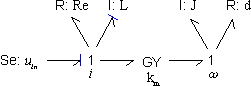4 Apply constraint causality. The 1 junction on the left has one bond with effort-out causality (seen from the 1 junction). The other bonds connected must therefore have effort-in causality (seen from the 1 junction). In a same way we can now assign causality for the GY-element: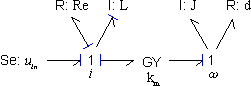5 Apply preferred causality. Only the right I-element is left. We can assign causality in its preferred form: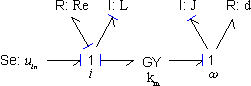6 Apply constraint causality. The 1 junction on the right has one bond with effort-out causality (seen from the 1 junction). The other bonds connected must therefore have effort-in causality. Therefore we can assign causality for the R-element: